# Use VLOOKUP Function as an alternative to a number of nested IF functions

In this article, you will learn how to use VLOOKUP function as an alternative to a number of Nested IF functions in Microsoft Excel.

VLOOKUP function is used to return a value from the data on the basis of common and unique lookup value from the leftmost column.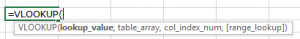Note: If we do not have the common value in other data from which we want to pick the data, then the formula will give the #N/A error. As you can see in the above-shown snapshot, the employee code L1089 in not available in 1st data set so formula is displaying an error.

IFfunction is used to check if a condition is met and returns one value as True and another value as False.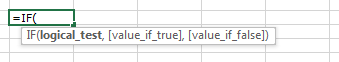Let’s take an example to understand how and where we can use Vlookup function instead of nested IF function.

In Excel, we have serial wise scores, column A contains serial numbers, and column B contains scores. We have a drop list of serial numbers in cell C2. We want if we select any number from the drop down list, cell D2 shows us the scores for that serial number.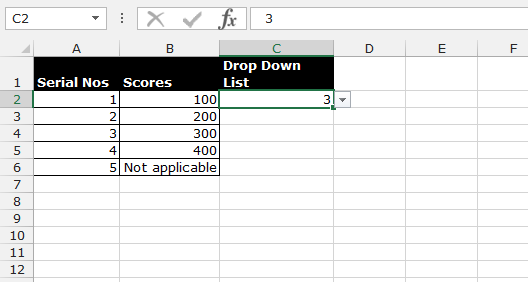First we will try through If function, follow below given steps:-

• Write the formula in cell D2.
• =IF(C2=1,100,IF(C2=2,200,IF(C2=3,300,IF(C2=4,400,"Not available"))))
• Press Enter on your keyboard.
• The function will return the score as per the number available in cell C2.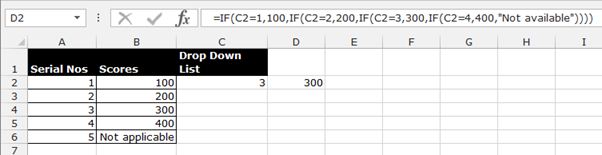• If cell C2 contains 1 number, then the formula of cell D2 will return 100, if cell C2 contains 2 numbers, then the formula will return 200.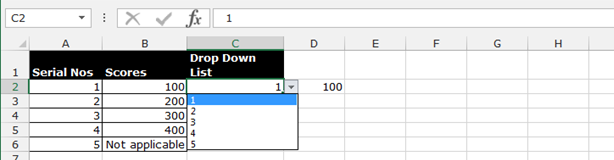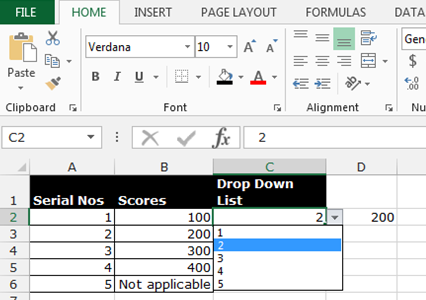Now, let’s use VLOOKUP function:-

• Write the formula in cell D2.
• =VLOOKUP(C2,A2:B6,2,FALSE)
• Press Enter on your keyboard.
• The function will return the score as per the number available in cell C2.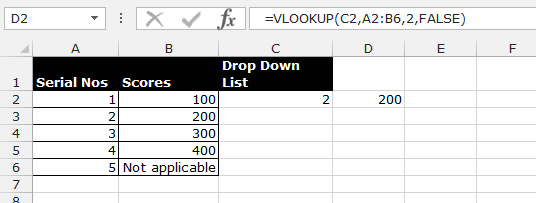• If the cell C2 contains 4 numbers, then the formula of cell D2 will return 100, if cell C2 contains 5 numbers, formula will return “Not Applicable”.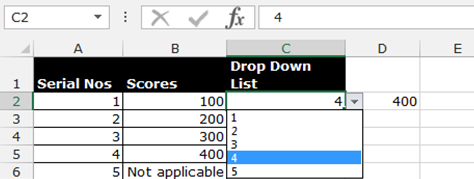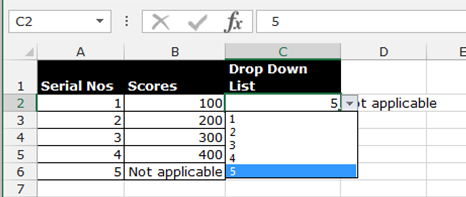Note: - This alternative will work only for lesser data, because in a cell, we are allowed to put the If condition 7 times only.